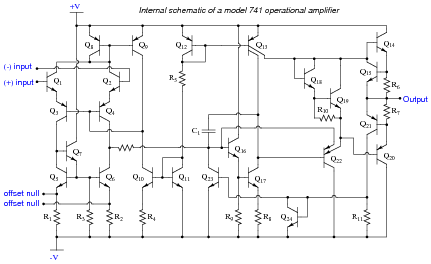# Active Loads in Amplifier Circuits

## Discrete Semiconductor Devices and Circuits

• #### Question 1

We know that the current in a series circuit may be calculated with this formula:

$$I=\frac{E_{total}}{R_{total}}$$

We also know that the voltage dropped across any single resistor in a series circuit may be calculated with this formula:

$$E_R=IR$$

Combine these two formulae into one, in such a way that the I variable is eliminated, leaving only ER expressed in terms of Etotal, Rtotal, and R.

• #### Question 2

Determine what will happen to the output voltage (Vout) and resistor R1‘s current (IR1) in this circuit as the resistance of R2 is increased: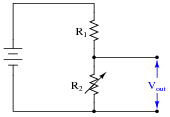• #### Question 3

Suppose we were to compare the performance of two voltage divider circuits side-by-side. The circuit on the left has one variable resistor (R2), while the circuit on the right has two variable resistors (R1 and R2). The right-hand circuit’s resistors are ganged together in such a way that as one resistance increases, the other will decrease by the same amount, keeping the circuit’s total resistance constant: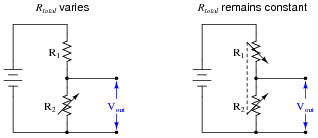Knowing that the voltage output by a voltage divider is described by the following formula, determine which voltage divider circuit yields the greatest change in output voltage for a given change in R2‘s resistance.

$$V_{out}=V_{battery}(\frac{R_2}{R_1+R_2})$$

• #### Question 4

The purpose of a current mirror circuit is to maintain constant current through a load despite changes in that load’s resistance: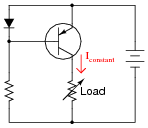If we were to crudely model the transistor’s behavior as an automatically-varied rheostat - constantly adjusting resistance as necessary to keep load current constant - how would you describe this rheostat’s response to changes in load resistance?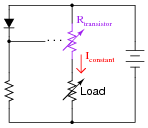In other words, as Rload increases, what does Rtransistor do - increase resistance, decrease resistance, or remain the same resistance it was before? How does the changing value of Rtransistor affect total circuit resistance?

• #### Question 5

An interesting technique to achieve extremely high voltage gain from a single-stage transistor amplifier is to substitute an active load for the customary load resistor (located at the collector terminal):Usually, this “active load” takes the form of a current mirror circuit, behaving as a current regulator rather than as a true current source.

Explain why the presence of an active load results in significantly more voltage gain than a plain (passive) resistor. If the active load were a perfect current regulator, holding collector current absolutely constant despite any change in collector-base conductivity for the main amplifying transistor, what would the voltage gain be?

• #### Question 6

Identify as many active loads as you can in the following (simplified) schematic of an LM324 operational amplifier circuit: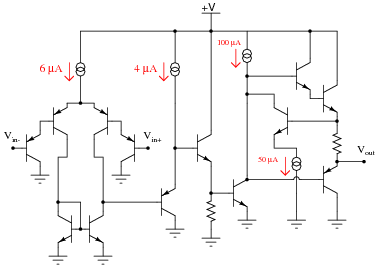• #### Question 7

Identify as many active loads as you can in the following schematic of an LM741 operational amplifier circuit,along with their respective (amplifying) transistors: